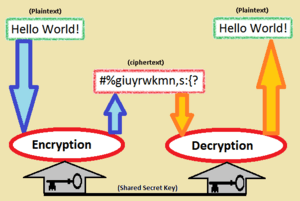# Symmetric-key algorithm facts for kids

Kids Encyclopedia FactsIn a symmetric-key algorithm the key used to encrypt is the same as the one used to decrypt. For this reason, it needs to be kept secret.

Symmetric-key algorithms is a method in cryptography. It is when the keys for decryption and encryption are exactly the same shared secret. You can generate the secret randomly, or from a password, or through a secret key-exchange procedure like Diffie-Hellman.

Symmetric-key algorithms are very important because they are faster on computers than the other kind:public-key algorithms. In public-key cryptography (asymmetric-key cryptography) the key for encryption can be given to the public with no problem, and everyone can send you secret messages. The key for encryption is "open" because, in practice, it cannot be used to get the key for decryption. This is very useful, but public-key cryptography algorithms are very slow on computers, so they are only used to send a secret key. Then symmetric-key algorithms are used for everything else because they are faster.

There are two kinds of symmetric-key algorithms, called stream ciphers and block ciphers. Stream ciphers encrypt a message as a stream of bits one at a time. Block ciphers take blocks of bits, encrypt them as a single unit, and sometimes use the answer later too. Blocks of 64 bits have been commonly used; though modern ciphers like the Advanced Encryption Standard use 128-bit blocks.

Examples of popular symmetric cyphers include Twofish, Serpent, AES (aka Rijndael), Blowfish, CAST5, RC4, TDES, and IDEA.

In history, some cryptanalysis methods exploited symmetry, so symmetric systems were less secure. Some attacks are called known-plaintext attacks, chosen plaintext attacks, differential cryptanalysis and linear cryptanalysis.

Other terms for symmetric-key encryption are secret-key, single-key, shared-key, one-key and eventually private-key encryption. This last term does not have the same meaning that the term private key has in public-key cryptography.

## Symmetric vs. asymmetric algorithms

Unlike symmetric algorithms, asymmetric key algorithms use a different key for encryption than for decryption. Meaning, a user knowing the encryption key of an asymmetric algorithm can encrypt messages, but cannot calculate the decryption key and cannot decrypt messages encrypted with that key. A short comparison of these two types of algorithms is given below:

### Speed

Symmetric-key algorithms are generally much less computationally intensive than asymmetric key algorithms. In practice, asymmetric key algorithms are typically hundreds to thousands times slower than symmetric key algorithms.

### Key management

One disadvantage of symmetric-key algorithms is the requirement of a shared secret key, with both parties holding the same copy at each end. In order to ensure secure communications between everyone in a group of n people a total of n(n - 1)/2 keys are needed, which is the total number of possible communication channels. To limit the impact of a potential discovery by a cryptographic attacker, they should be changed regularly and kept secure during distribution and in service. The process of selecting, distributing and storing keys is known as key management, and is difficult to achieve reliably and securely.

### Hybrid cryptosystem

In modern cryptosystems designs, both asymmetric (public key) and symmetric algorithms are used to take advantage of the best of both. Asymmetric algorithms are used to distribute symmetric-keys at the start of a session. Once a symmetric key is known to all parties of the session, faster symmetric-key algorithms using that key can be used to encrypt the remainder of the session. This simplifies the key distribution problem, because asymmetric keys only have to be distributed authentically, while symmetric keys need to be distributed in both an authentic and confidential manner.

Systems that use such a hybrid approach include SSL, PGP and GPG, etc.

## Related pagesSymmetric-key algorithm Facts for Kids. Kiddle Encyclopedia.